# Physics Practicals For Class 12

Physics is one of the most important subjects in Class 12. As the CBSE exam approaches, students get busy preparing for different subjects. But an essential part of the CBSE exam is the practical exams which consist of 30 marks.

Students must know all the experiments along with theorems, laws, and numerical to understand all the concepts of 12th standard physics in a detailed way. Two experiments (8 + 8 marks) are asked from each section in the practical exam. The experiment records and activities consist of 6 marks, the project has 3 marks and viva on the experiment consist of 5 marks.

The Physics Practicals For Class 12 CBSE is given here so that students can understand the experiments in a better way. Students are suggested to study the theory and law behind the experiment properly before performing the experiment.

 Class 12 Physics Experiments Section A 1. To determine resistance per cm of a given wire by plotting a graph for potential difference versus current. 2.To find resistance of a given wire using metre bridge and hence determine the resistivity (specific resistance) of its material. 3. To verify the laws of combination (series) of resistances using a metre bridge. 4. To verify the Laws of combination (parallel) of resistances using a metre bridge. 5. To compare the EMF of two given primary cells using potentiometer. 6. To determine the internal resistance of given primary cell using potentiometer. 7. To determine resistance of a galvanometer by half-deflection method and to find its figure of merit. 8. To convert the given galvanometer (of known resistance and figure of merit) into a voltmeter of desired range and to verify the same. 9. To convert the given galvanometer (of known resistance and figure of merit) into an ammeter of desired range and to verify the same. 10. To find the frequency of AC mains with a sonometer. Section B 1. To find the value of v for different values of u in case of a concave mirror and to rind the focal length. 2. To find the focal Length of a convex mirror, using a convex lens. 3. To find the focal Length of a convex lens by plotting graphs between u and v or between 1/u and 1/v. 4. To find the focal Length of a concave lens, using a convex lens. 5. To determine angle of minimum deviation for a given prism by plotting a graph between angle of incidence and angle of deviation. 6. To determine refractive index of a glass slab using a travelling microscope. 7. To find refractive index of a liquid by using convex Lens and plane mirror. 8. To draw the I-V characteristic curve for a p-n junction in forward bias and reverse bias. 9. To draw the characteristic curve of a Zener diode and to determine its reverse break down voltage. 10. To study the characteristic of a common – emitter npn or pnp transistor and to find out the values of current and voltage gains.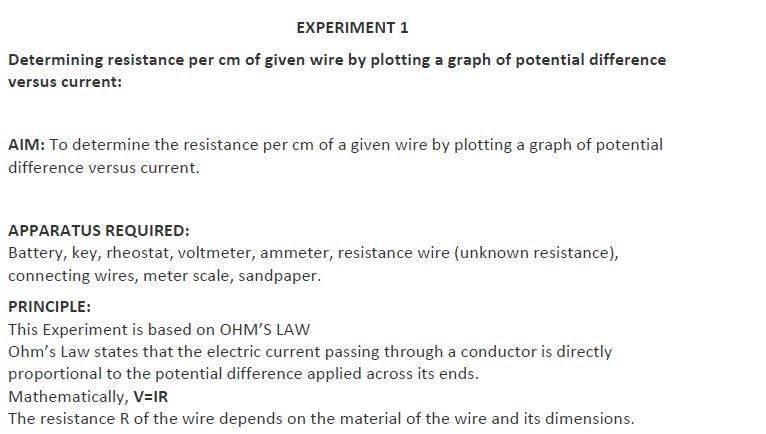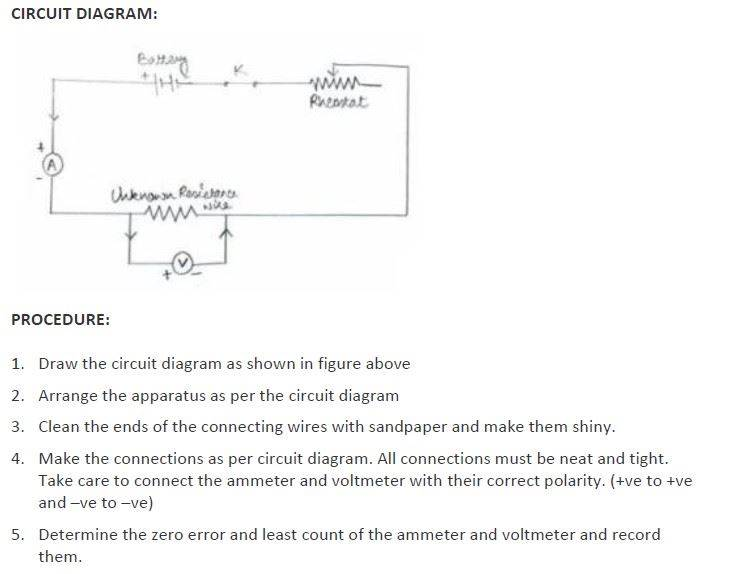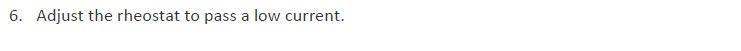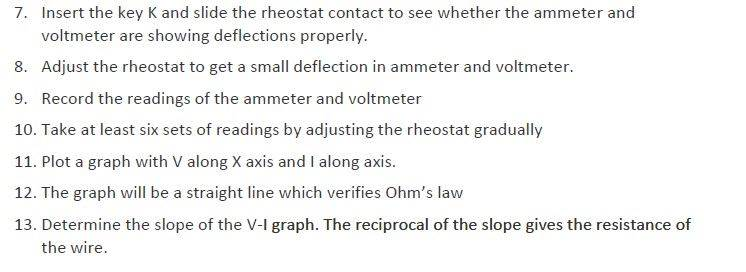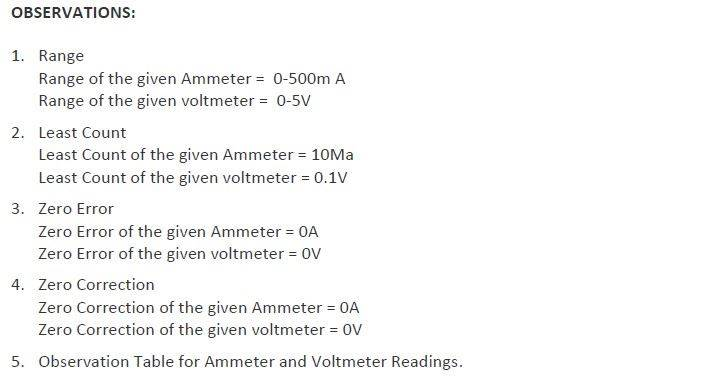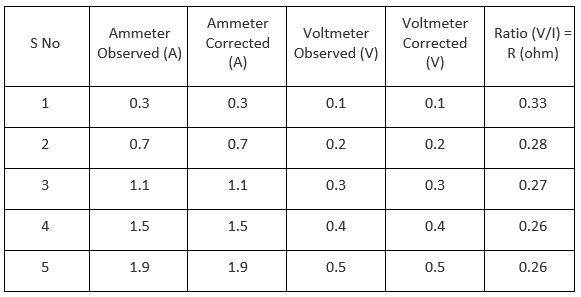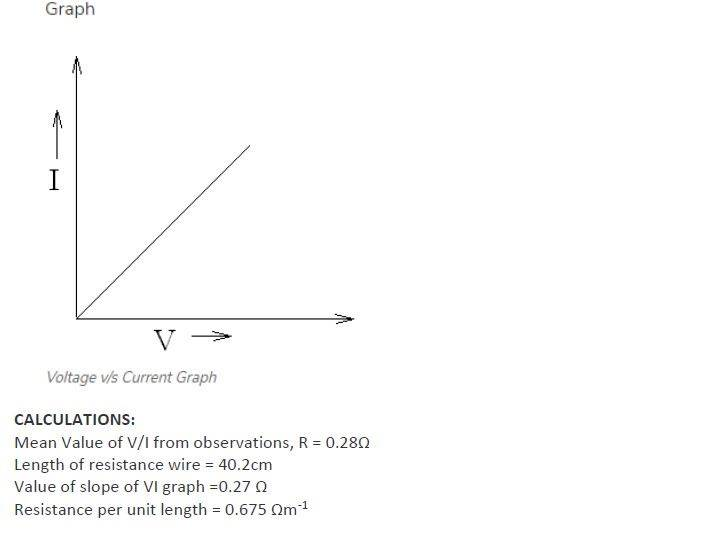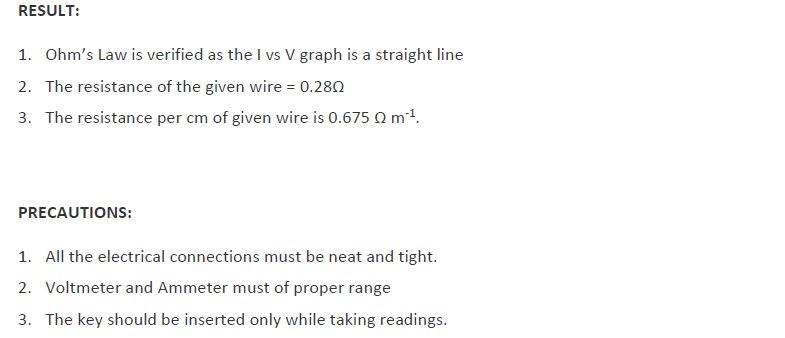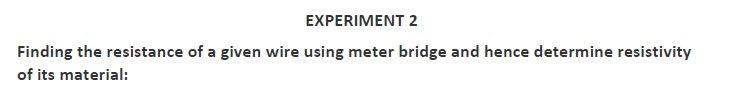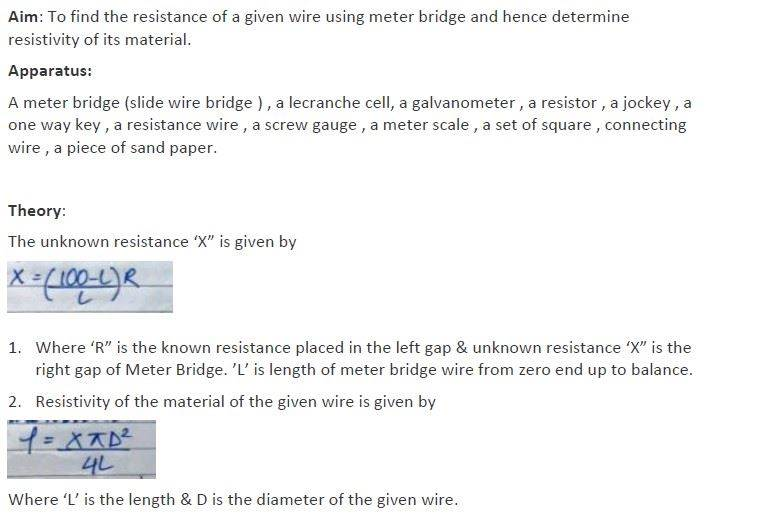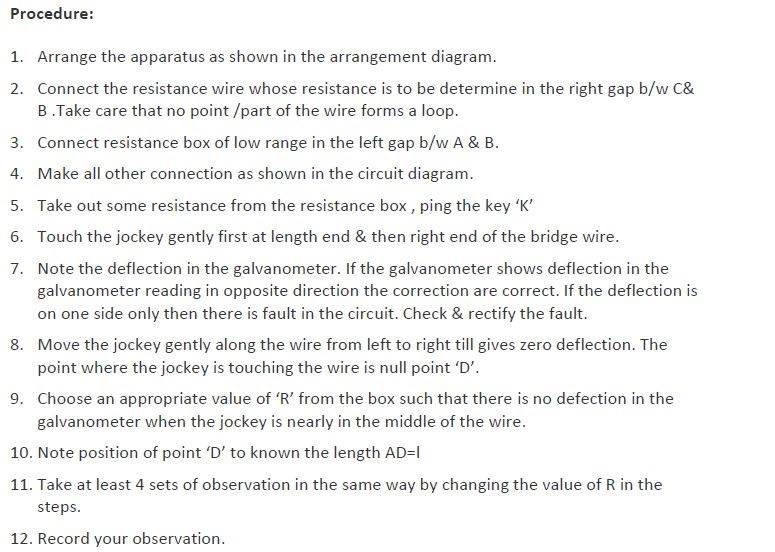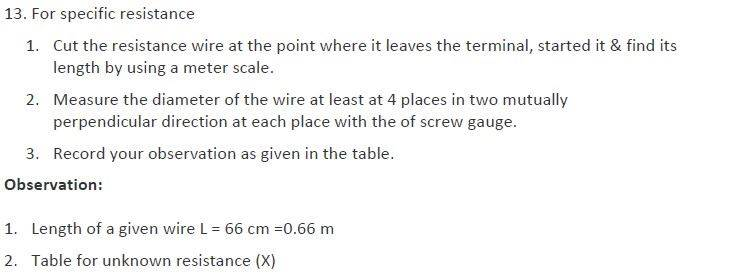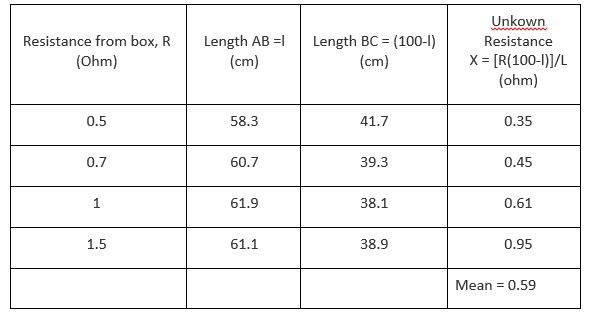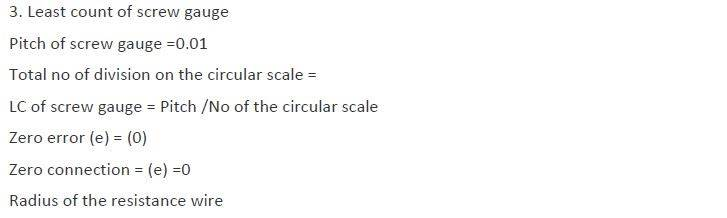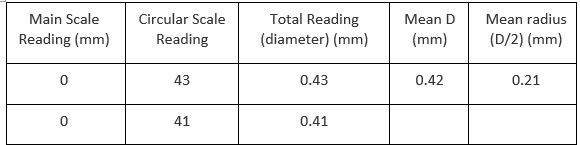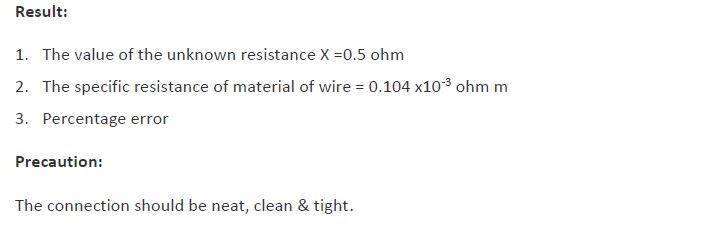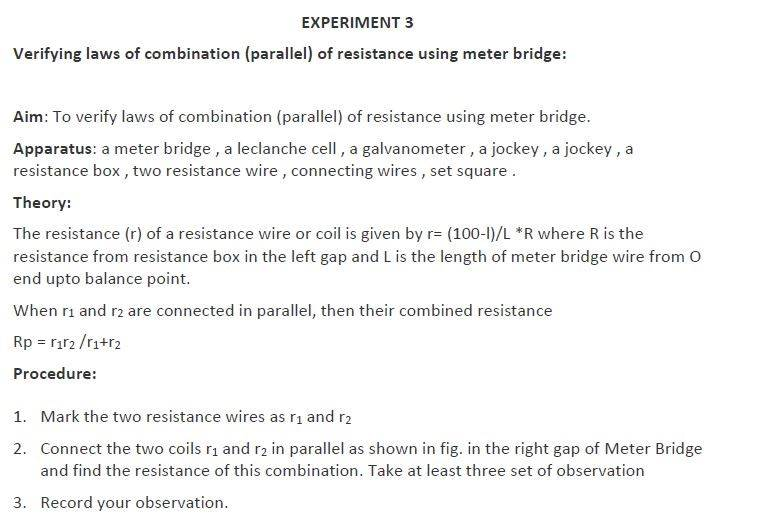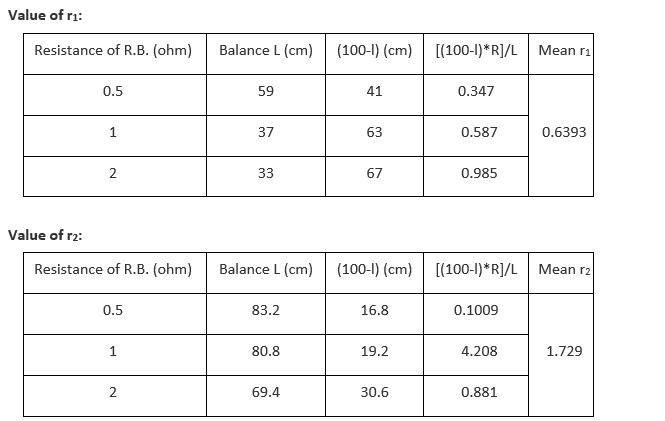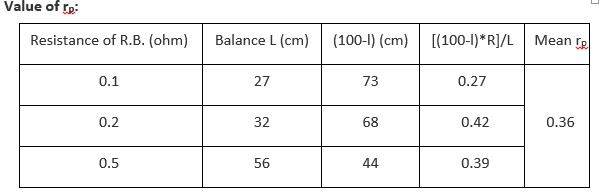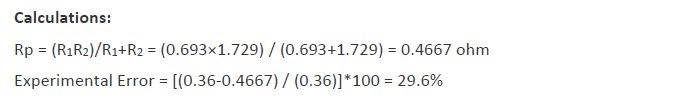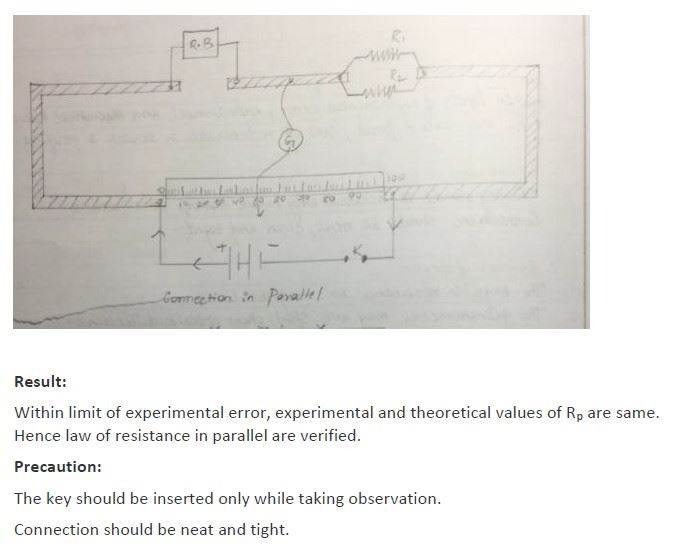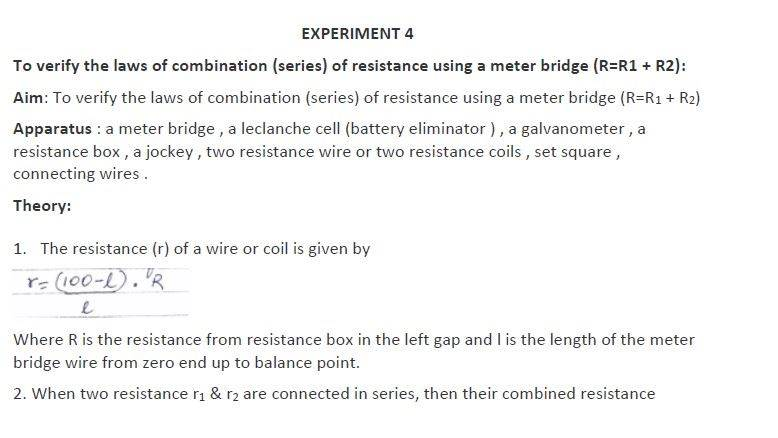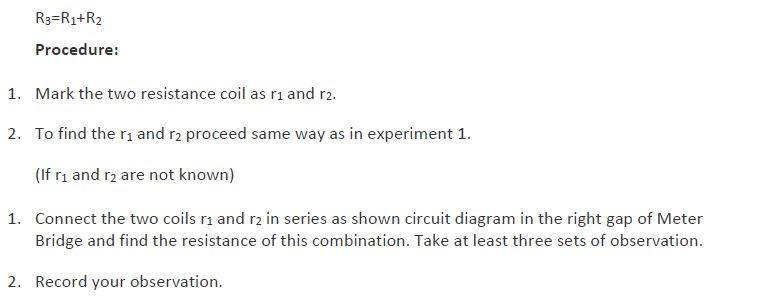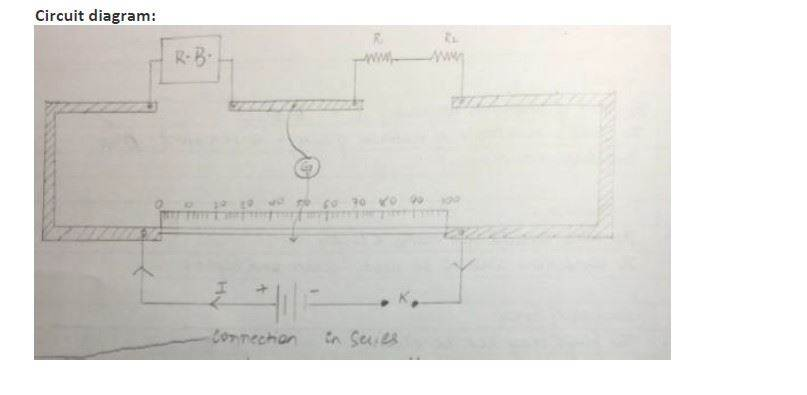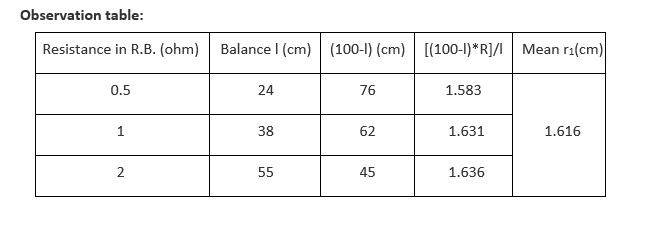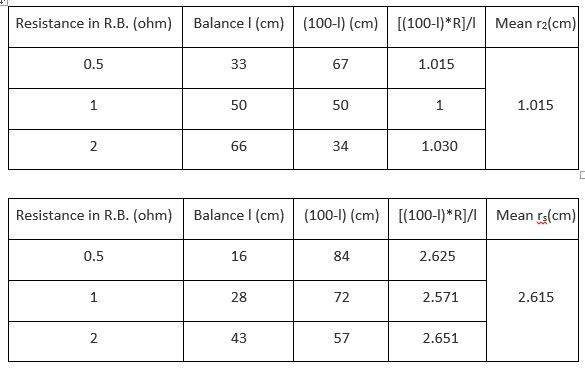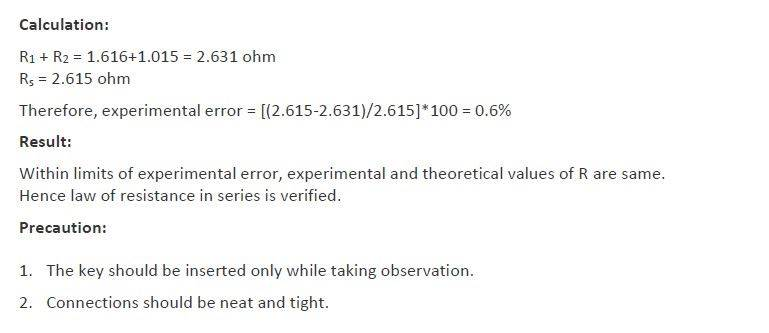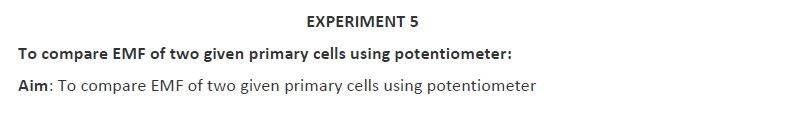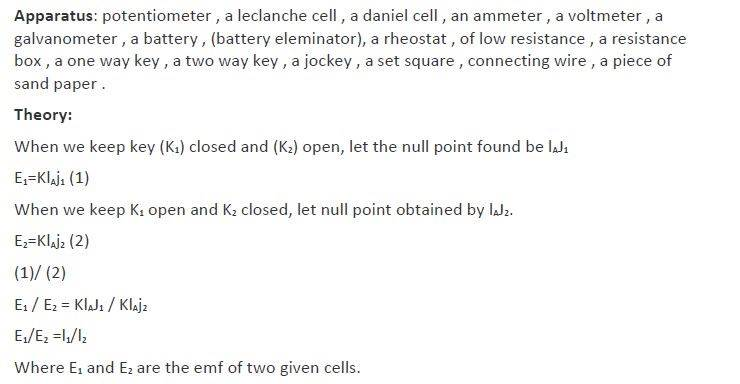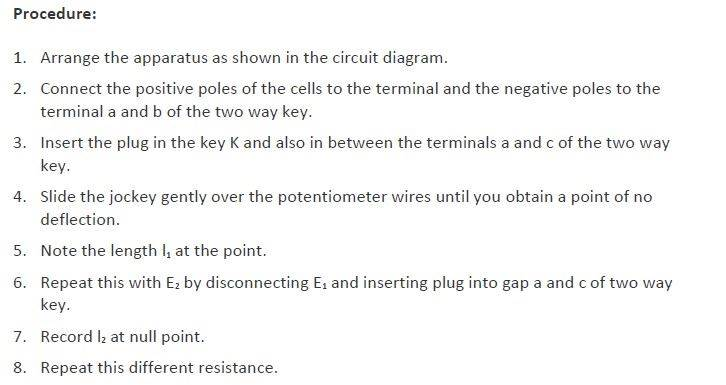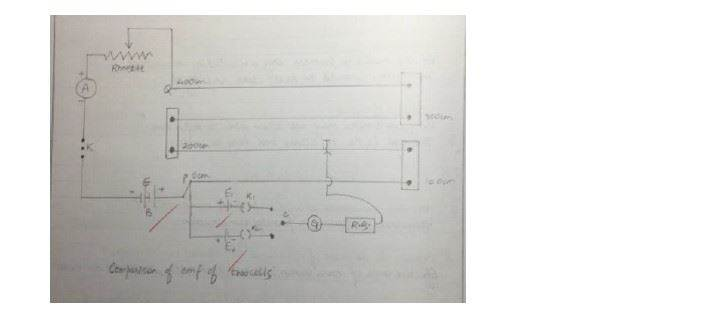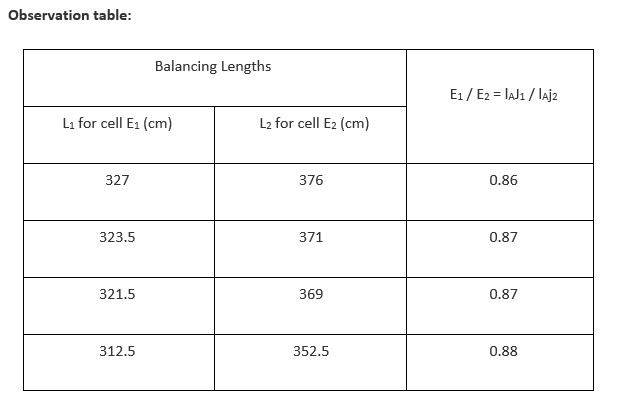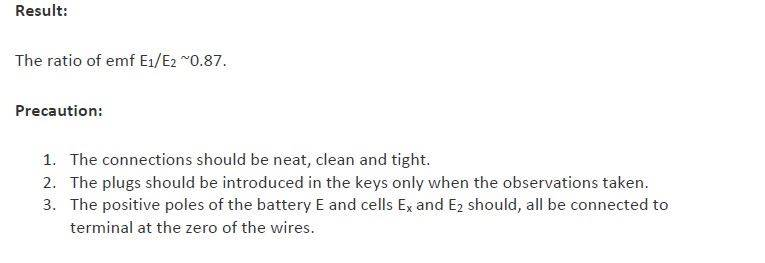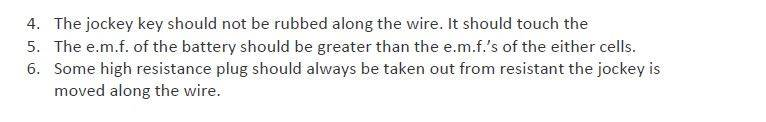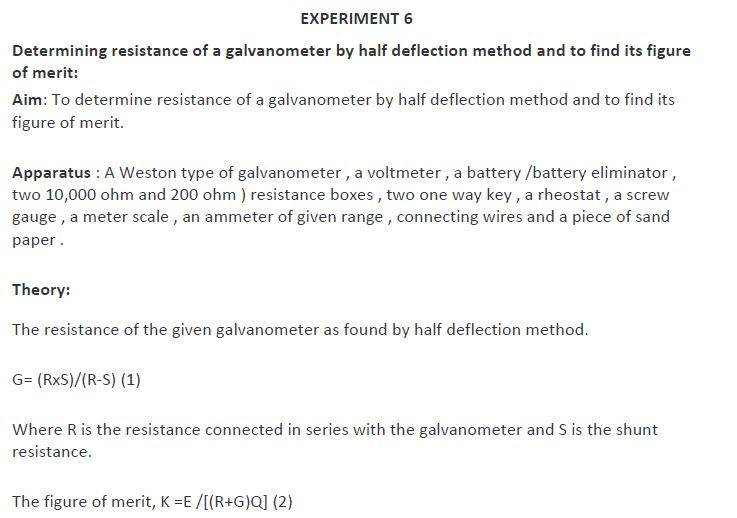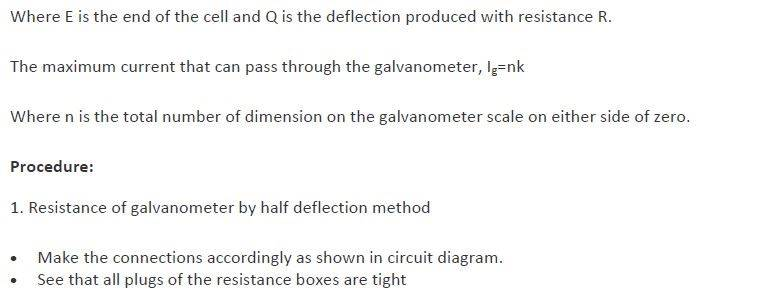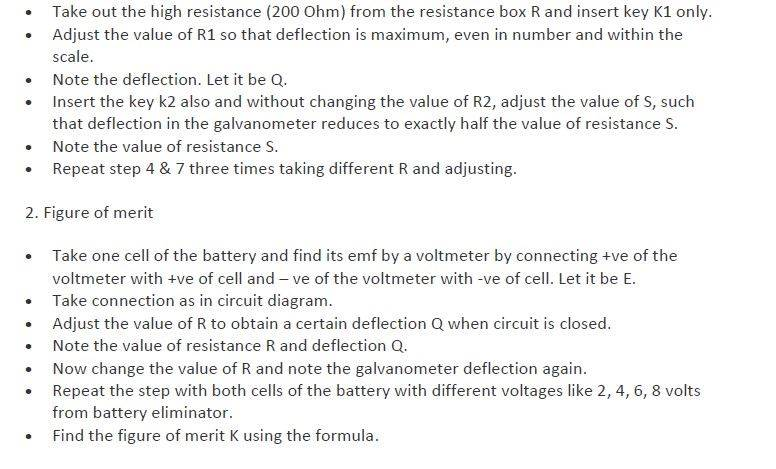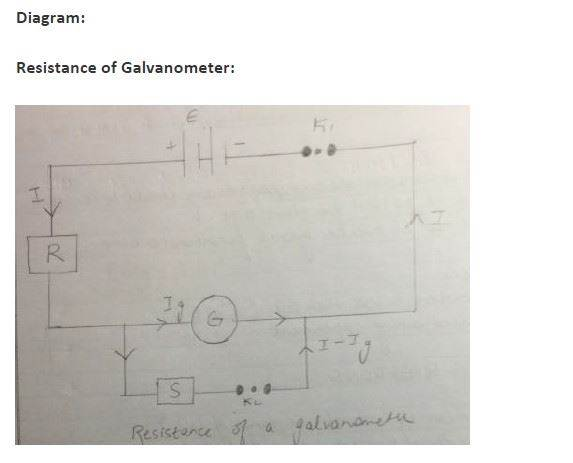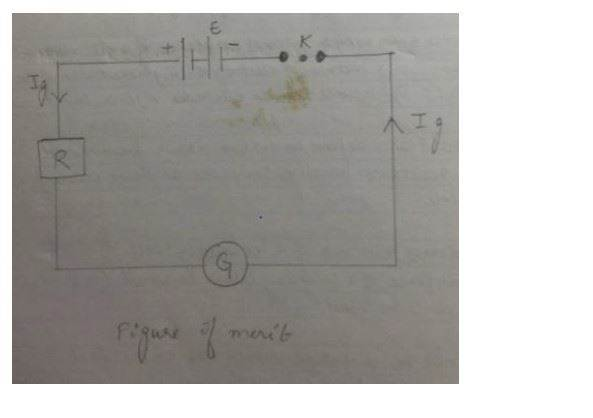Observation table :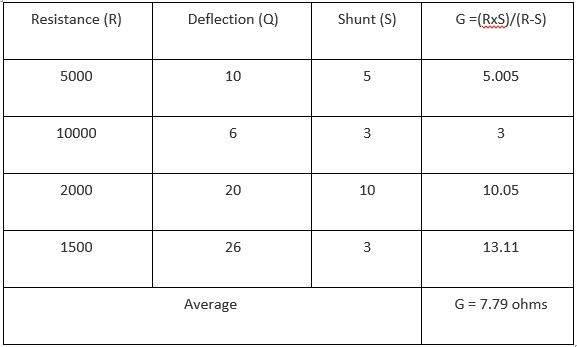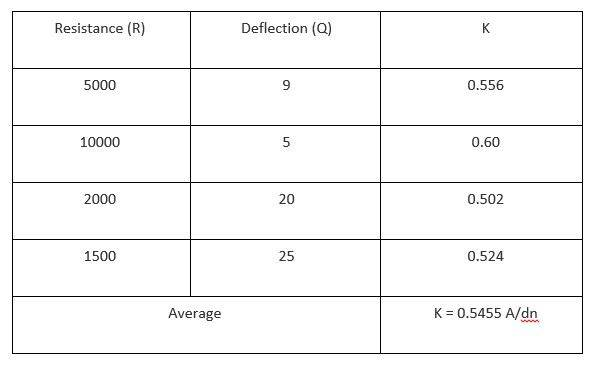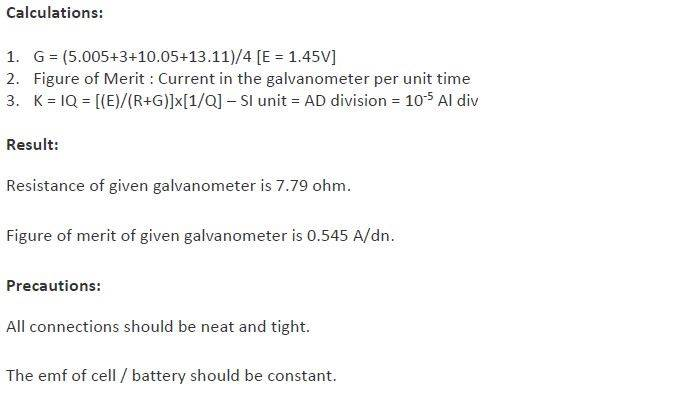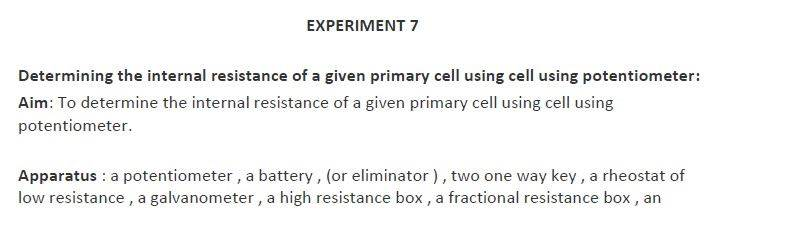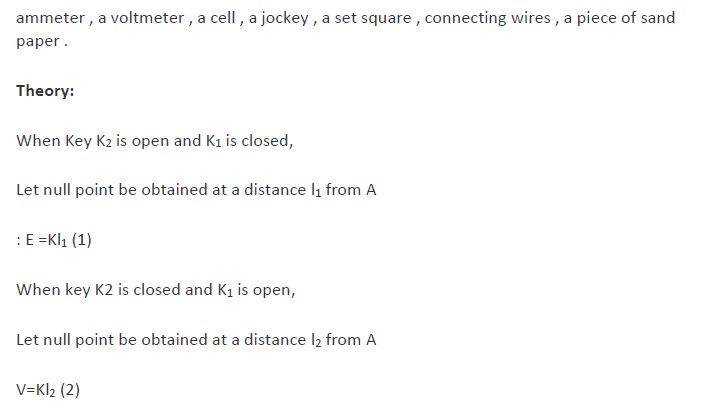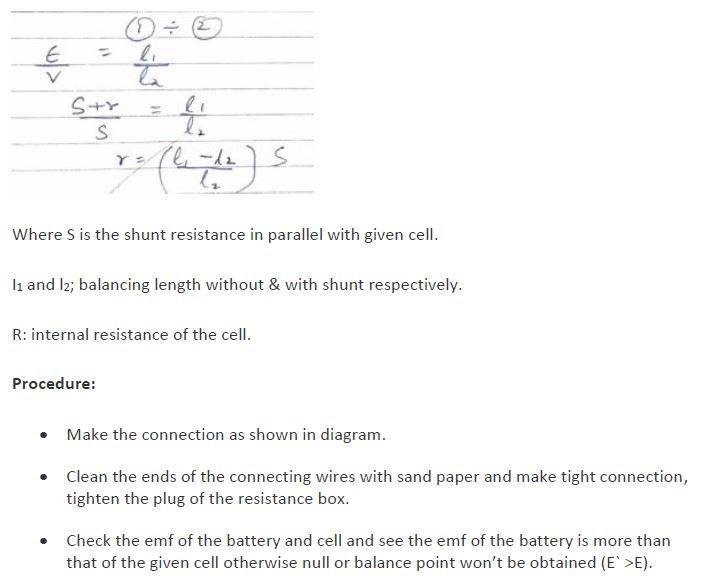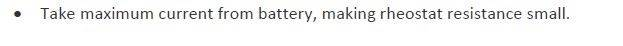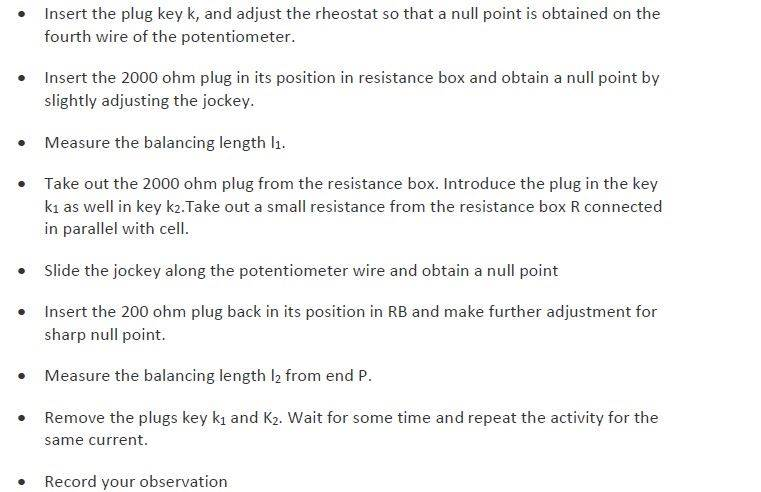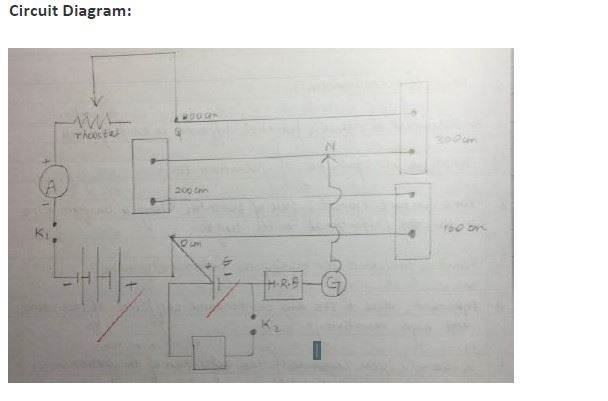Observation table :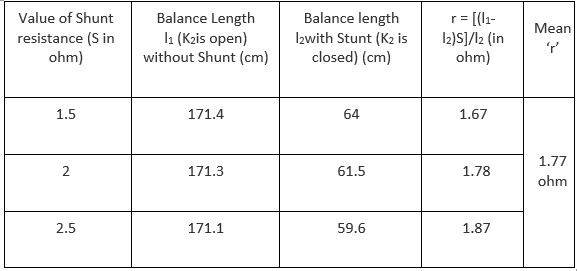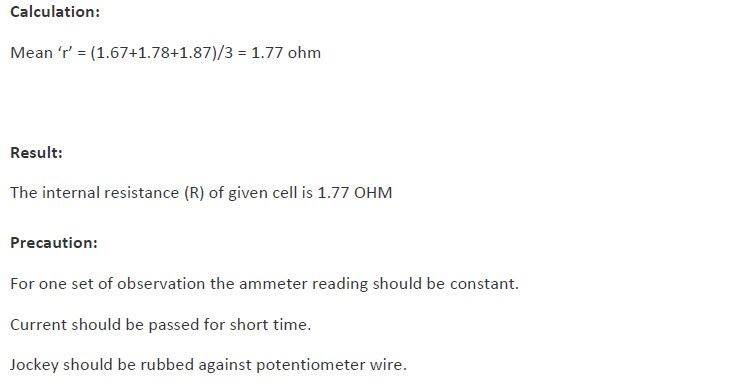Stay tuned with Byju’s to get the latest notification on CBSE along with CBSE syllabus , sample papers, marking scheme and more.

 NCERT Related Articles NCERT Solutions for Class 12 NCERT Solutions for Class 11 NCERT Solutions for Class 10 NCERT Solutions for Class 12 Maths NCERT Solutions for Class 11 Maths NCERT Solutions for Class 10 Maths NCERT Solutions for Class 12 Physics NCERT Solutions for Class 11 Physics NCERT Solutions for Class 10 Science NCERT Solutions for Class 12 Chemistry NCERT Solutions for Class 11 Chemistry NCERT Solutions for Class 6 NCERT Solutions for Class 12 Biology NCERT Solutions for Class 11 Biology NCERT Solutions for Class 6 Maths NCERT Solutions for Class 9 NCERT Solutions for Class 8 NCERT Solutions for Class 6 Science NCERT Solutions for Class 9 Maths NCERT Solutions for Class 8 Maths NCERT Solutions for Class 4 NCERT Solutions for Class 9 Science NCERT Solutions for Class 8 Science NCERT Solutions for Class 4 Maths NCERT Solutions for Class 5 NCERT Solutions for Class 7 NCERT Solutions for Class 7 Maths NCERT Solutions for Class 4 Science NCERT Solutions for Class 5 Maths NCERT Solutions for Class 7 Science NCERT Solutions NCERT Books NCERT Solutions for Class 5 Science